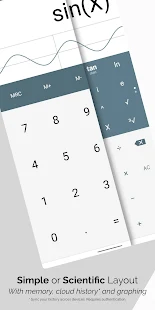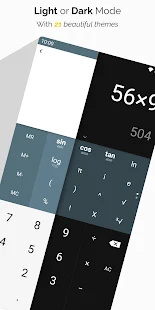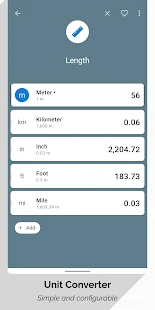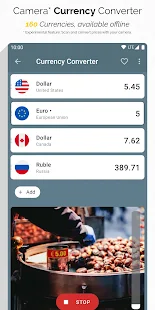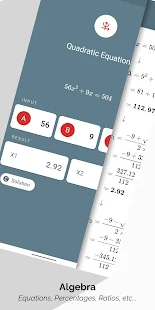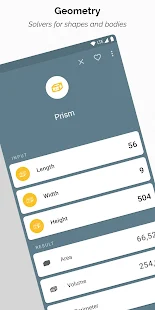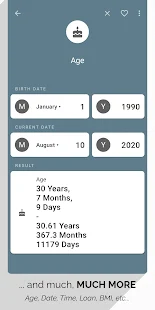Descriptions :

The original all-in-one calculator for Android It is a FREE, comprehensive and easy-to-use multiple calculator and converter. Designed with simplicity in mind, it helps you solve everyday problems. From simple or complex calculations, to unit and currency conversions, percentages. , proportions, areas, volumes, etc ... it does it all. And it does it well! Passionate development combined with constant feedback we receive from our users resulted in what we believe to be the best multiple calculator in the store.With over 75 FREE calculators and unit converters packed with a simple or scientific calculator, it is the only calculator that you will need from now on on your device.

Features:
- Percentage calculator
- Proportion calculator
- Proportion calculator
- Arithmetic, geometric and harmonic means calculator
- Solver of equations linear, quadratic and system of equations.
- Combinations and permutations
- Decimal to fraction
- Fraction Simplifier
- Prime number checker
- Highest common factor and lowest common multiples calculator
- Random number generator

Required Android Versions : Lollipop [5.0–5.0.2] - Marshmallow [6.0 - 6.0.1] - Nougat [7.0 – 7.1.1] - Oreo [8.0-8.1]

## Download All-In-One Calculator Pro V 2.1.5 APK Mod Free

All-in-One_Calculator_Pro_v2.1.5_Mod_Plus.apk